Author: Oscar Cronquist Article last updated on May 04, 2022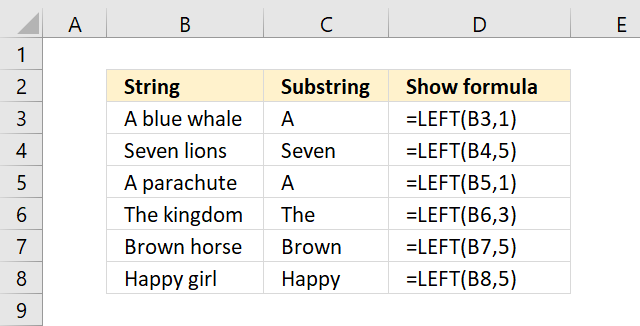The LEFT function extracts a specific number of characters always starting from the left.

Formula in cell C3:

=LEFT(B3, 1)

The formula in cell C3 demonstrated in the image above extracts the first letter from the value in cell B3.

## 1. Excel Function Syntax

The LEFT function has two arguments, the second argument is optional. It defaults to 1 meaning the first character from the left is extracted if the second argument is omitted.

LEFT(text, [num_chars])

## 2. LEFT Function Arguments

 text Text string or a cell reference to a text string. [num_chars] The number of characters to extract. Optional. If this argument is not entered only the first character is extracted.

## 3. LEFT function example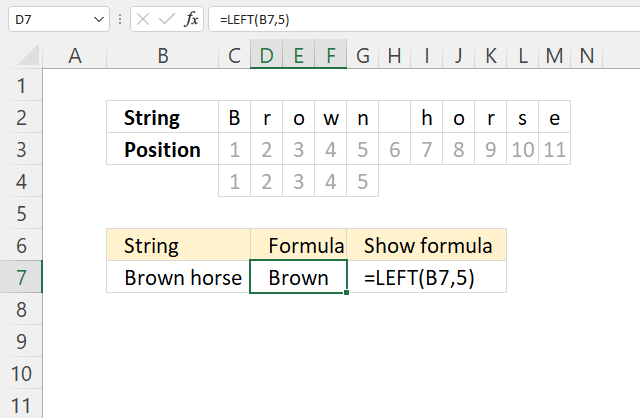The image above shows the string "Brown horse", each cell is numbered from 1 to 11.

The formula =LEFT(B7, 5) extracts the five first characters from the left which is "Brown".

## 4. LEFT until character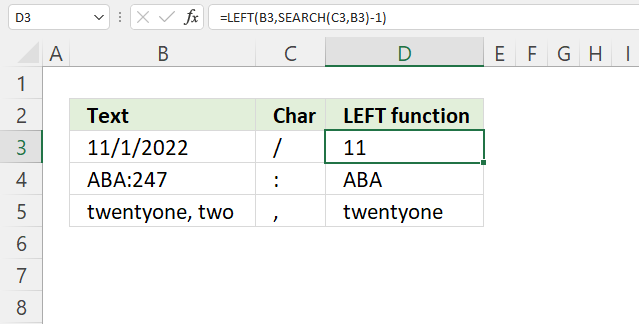The image above demonstrates a formula in cell D3 that extracts characters from left until a specified character is found. In this case / (forward slash).

Formula in cell D3:

=LEFT(B3, SEARCH(C3, B3)-1)

### Explaining formula

#### Step 1 - Search string for given character

The SEARCH function returns a number representing the position of character at which a specific text string is found reading left to right.

SEARCH(find_text,within_text, [start_num])

SEARCH(C3, B3)

becomes

SEARCH("/","11/1/2022")

and returns 3.

#### Step 2 - Subtract with 1

The minus character lets you subtract numbers in an Excel formula.

SEARCH(C3, B3)-1

becomes

3-1 equals 2.

#### Step 3 - Extract given number of characters from left

LEFT(B3, SEARCH(C3, B3)-1)

becomes

LEFT(B3, 2)

becomes

LEFT("11/1/2022", 2)

and returns "11".

## 5. LEFT function until space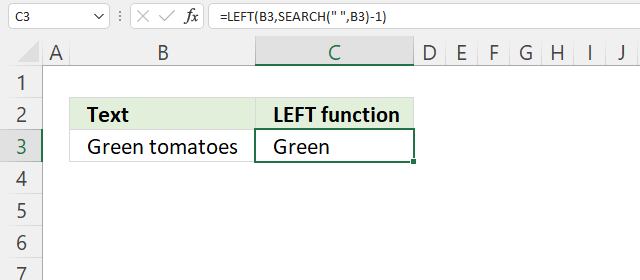Formula in cell C3:

=LEFT(B3, SEARCH(" ", B3)-1)

### Explaining formula

#### Step 1 - Find space character in string

The SEARCH function returns a number representing the position of character at which a specific text string is found reading left to right.

SEARCH(find_text,within_text, [start_num])

SEARCH(" ", B3)

becomes

SEARCH(" ","Green tomatoes")

and returns 6. The space character is the sixth character in the string.

#### Step 2 - Subtract number with 1

The minus character lets you subtract numbers in an Excel formula.

SEARCH(" ", B3)-1

becomes

6-1 equals 5.

#### Step 3 - Extract a given number of characters from left

LEFT(B3, SEARCH(" ", B3)-1)

becomes

LEFT(B3, 5)

becomes

LEFT("Green tomatoes", 5)

and returns "Green".

## 6. LEFT function until second space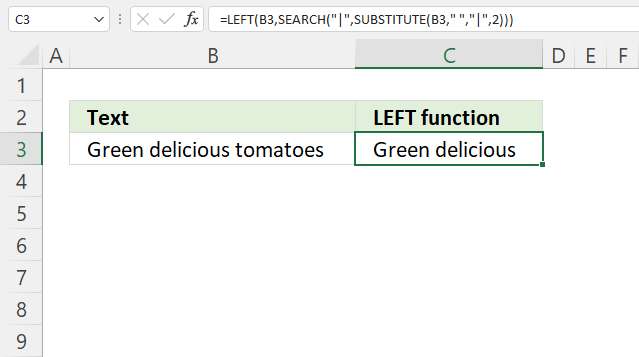The picture above shows a formula in cell C3 that extracts a string from cell B3 until the second space character from the left.

Formula in cell C3:

=LEFT(B3,SEARCH("|", SUBSTITUTE(B3," ","|",2)))

### Explaining formula

#### Step 1 - Replace second space character

The SUBSTITUTE function allows you to replace a given string of characters, it also lets you choose which instance. I am using a character not being used in the string in this case |

SUBSTITUTE(textold_textnew_text, [instance_num])

SUBSTITUTE(B3," ","|",2)

becomes

SUBSTITUTE("Green delicious tomatoes"," ","|",2)

and returns "Green delicious|tomatoes". The second space character is now |.

#### Step 2 - Find position of character

The SEARCH function returns a number representing the position of character at which a specific text string is found reading left to right.

SEARCH(find_text,within_text, [start_num])

SEARCH("|", SUBSTITUTE(B3," ","|",2))

becomes

SEARCH("|", "Green delicious|tomatoes")

and returns 16.

#### Step 3 - Extract a given number of characters from left

LEFT(B3,SEARCH("|", SUBSTITUTE(B3," ","|",2)))

becomes

LEFT(B3, 16)

becomes

LEFT("Green delicious tomatoes", 16)

and returns "Green delicious".

## 7. LEFT until dash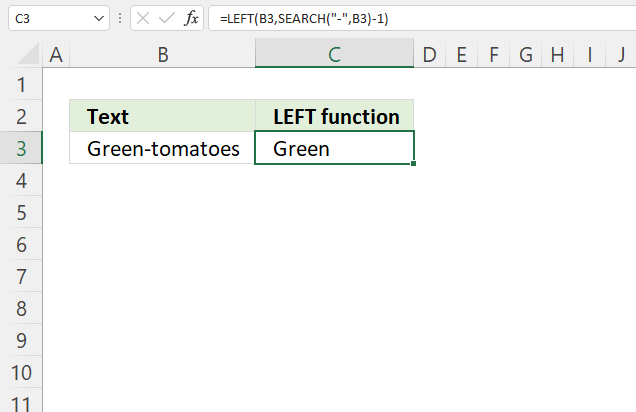The following formula extracts characters until a dash character.

Formula in cell C3:

=LEFT(B3, SEARCH("-", B3)-1)

### Explaining formula

#### Step 1 - Find the position of dash character

The SEARCH function returns a number representing the position of character at which a specific text string is found reading left to right.

SEARCH(find_text,within_text, [start_num])

SEARCH("-", B3)

becomes

SEARCH("-", "Green-tomatoes")

and returns 6.

#### Step 2 - Subtract with 1

The minus character lets you subtract numbers in an Excel formula.

SEARCH("-", B3)-1

becomes

6-1 equals 5.

#### Step 3 - Extract a given number of characters from left

LEFT(B3, SEARCH("-", B3)-1)

becomes

LEFT(B3, 5)

becomes

LEFT("Green-tomatoes", 5)

and returns "Green".

## 8. LEFT until number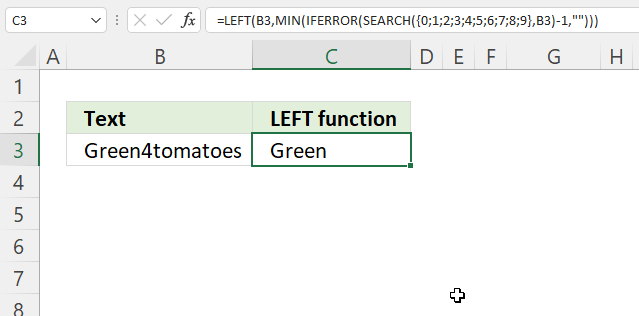Array formula in cell C3:

=LEFT(B3, MIN(IFERROR(SEARCH({0; 1; 2; 3; 4; 5; 6; 7; 8; 9}, B3)-1, "")))

### 8.1 How to enter an array formula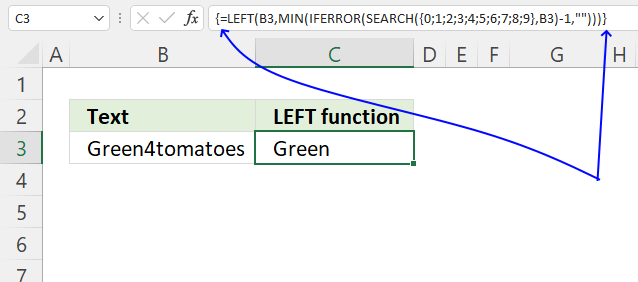Excel 365 users can ignore these instructions, enter the formula as a regular formula.

1. Copy array formula.
2. Double press on cell C3, a prompt appears.
3. Paste array formula to the cell.
4. Press and hold CTRL + SHIFT keys simultaneously.
5. Press Enter once.
6. Release all keys.

A beginning and ending curly bracket is now shown, don't enter these characters yourself. They appear automatically, see the image above.

### 8.2 Explaining array formula

#### Step 1 - Search for numbers between 0 (zero) and 9

The SEARCH function returns a number representing the position of character at which a specific text string is found reading left to right.

SEARCH(find_text,within_text, [start_num])

SEARCH({0; 1; 2; 3; 4; 5; 6; 7; 8; 9}, B3)

becomes

SEARCH({0; 1; 2; 3; 4; 5; 6; 7; 8; 9}, "Green4tomatoes")

and returns

{#VALUE!; #VALUE!; #VALUE!; #VALUE!; 6; #VALUE!; #VALUE!; #VALUE!; #VALUE!; #VALUE!}.

The SEARCH function returns #VALUE! error if nothing found.

#### Step 2 - Subtract with 1

The minus character lets you subtract numbers in an Excel formula.

SEARCH({0; 1; 2; 3; 4; 5; 6; 7; 8; 9}, B3)-1

becomes

{#VALUE!; #VALUE!; #VALUE!; #VALUE!; 6; #VALUE!; #VALUE!; #VALUE!; #VALUE!; #VALUE!}-1

and returns

{#VALUE!; #VALUE!; #VALUE!; #VALUE!; 5; #VALUE!; #VALUE!; #VALUE!; #VALUE!; #VALUE!}

#### Step 3 - Handle errors

The IFERROR function lets you catch most errors in Excel formulas, it returns TRUE if value is an error and FALSE if not.

IFERROR(valuevalue_if_error)

IFERROR(SEARCH({0; 1; 2; 3; 4; 5; 6; 7; 8; 9}, B3)-1, "")

becomes

IFERROR({#VALUE!; #VALUE!; #VALUE!; #VALUE!; 5; #VALUE!; #VALUE!; #VALUE!; #VALUE!; #VALUE!}, "")

and returns

{""; ""; ""; ""; 5; ""; ""; ""; ""; ""}.

#### Step 4 - Extrcat smallest number

The MIN function returns the minimum number in a cell range or array, it ignores text and blank values.

MIN(number1, [number2], ...)

MIN(IFERROR(SEARCH({0; 1; 2; 3; 4; 5; 6; 7; 8; 9}, B3)-1, ""))

becomes

MIN({""; ""; ""; ""; 5; ""; ""; ""; ""; ""})

and returns 5.

#### Step 5 -

LEFT(B3, MIN(IFERROR(SEARCH({0; 1; 2; 3; 4; 5; 6; 7; 8; 9}, B3)-1, "")))

## 9. LEFT function remove characters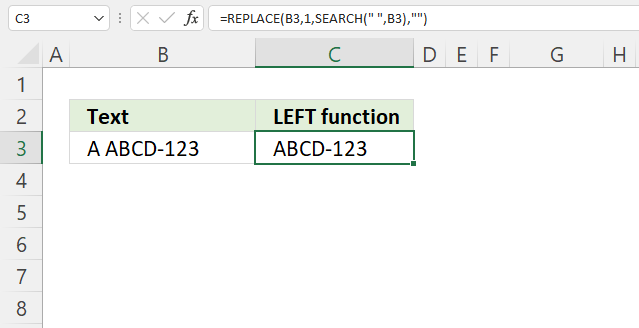The image above shows a formula in cell C3 that removes the first characters from the left until a space character is found.

A space character is found in the second position, the first and second character is removed.

Formula in cell C3:

=REPLACE(B3,1,SEARCH(" ",B3),"")

### Explaining formula

#### Step 1 - Find first space character

The SEARCH function returns a number representing the position of character at which a specific text string is found reading left to right.

SEARCH(find_text,within_text, [start_num])

SEARCH(" ",B3)

becomes

SEARCH(" ","A ABCD-123")

and returns 2.

#### Step 2 - Replace characters with nothing

The REPLACE function replaces part of a text string, based on the character position, and the number of characters, with a different text string.

REPLACE(old_text, start_num, num_chars, new_text)

REPLACE(B3,1,SEARCH(" ",B3),"")

becomes

REPLACE(B3,1,2,"")

becomes

REPLACE("A ABCD-123", 1, 2,"")

and returns "ABCD-123".

## 10. LEFT function for numbers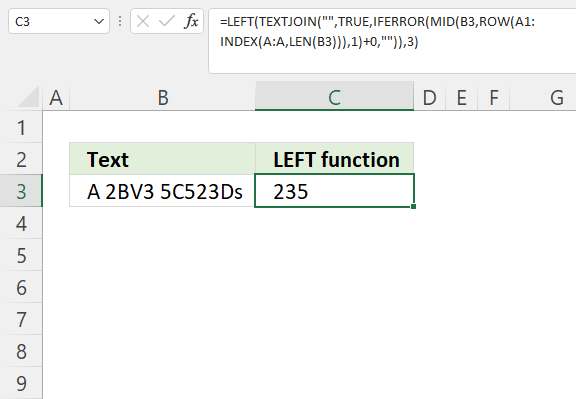The formula in cell C3 extracts the three first numbers in cell B3, note that there are other characters than numbers between the numbers as well.

Array formula in cell C3:

=LEFT(TEXTJOIN("",TRUE,IFERROR(MID(B3,ROW(A1:INDEX(A:A,LEN(B3))),1)+0,"")),3)

How to enter an array formula

### 10.1 Explaining formula

#### Step 1 - Count characters

The LEN function calculates the number of characters in a value.

LEN(value)

LEN(B3)

becomes

LEN("A 2BV3 5C523Ds")

and returns 14.

#### Step 2 - Create a cell range containing as many rows as there are characters in cell B3

The INDEX function returns a value based on a row and column number, however, it can also build a cell reference.

A1:INDEX(A:A,LEN(B3))

becomes

A1:INDEX(A:A,14)

and returns A1:A14.

#### Step 3 - Create an array of row numbers

The ROW function returns a number representing the row based on a cell reference. It also returns an array of row numbers if a cell reference containing multiple rows is used.

ROW(A1:INDEX(A:A,LEN(B3)))

becomes

ROW(A1:A14)

and returns {1; 2; 3; 4; 5; 6; 7; 8; 9; 10; 11; 12; 13; 14}.

#### Step 4 - Split value in cell B3 to an array containing a character each

The MID function returns a substring from a string based on the starting position and the number of characters you want to extract.

MID(text, start_num, num_chars)

MID(B3,ROW(A1:INDEX(A:A,LEN(B3))),1)

becomes

MID(B3, {1; 2; 3; 4; 5; 6; 7; 8; 9; 10; 11; 12; 13; 14}, 1)

becomes

MID("A 2BV3 5C523Ds", {1; 2; 3; 4; 5; 6; 7; 8; 9; 10; 11; 12; 13; 14}, 1)

and returns

{"A"; " "; "2"; "B"; "V"; "3"; " "; "5"; "C"; "5"; "2"; "3"; "D"; "s"}.

#### Step 5 - Add 0 (zero) to each character

Adding zero to each value allws us to identify if a value is a number or not.

MID(B3,ROW(A1:INDEX(A:A,LEN(B3))),1)+0

becomes

{"A"; " "; "2"; "B"; "V"; "3"; " "; "5"; "C"; "5"; "2"; "3"; "D"; "s"} + 0

and returns

{#VALUE!; #VALUE!; 2; #VALUE!; #VALUE!; 3; #VALUE!; 5; #VALUE!; 5; 2; 3; #VALUE!; #VALUE!}.

#### Step 6 - Replace error values with blanks

The IFERROR function handles errors in an Excel formula.

IFERROR(value, value_if_error)

IFERROR(MID(B3,ROW(A1:INDEX(A:A,LEN(B3))),1)+0,"")

becomes

IFERROR({#VALUE!; #VALUE!; 2; #VALUE!; #VALUE!; 3; #VALUE!; 5; #VALUE!; 5; 2; 3; #VALUE!; #VALUE!}, "")

and returns

{""; ""; 2; ""; ""; 3; ""; 5; ""; 5; 2; 3; ""; ""}.

Note that the error values are replaced with a blank "".

#### Step 7 - Join numbers

The TEXTJOIN function concatenates text strings.

TEXTJOIN(delimiterignore_emptytext1[text2], ...)

TEXTJOIN("",TRUE,IFERROR(MID(B3,ROW(A1:INDEX(A:A,LEN(B3))),1)+0,""))

becomes

TEXTJOIN("", TRUE, {""; ""; 2; ""; ""; 3; ""; 5; ""; 5; 2; 3; ""; ""})

and returns "235523".

#### Step 8 - Extract first three numbers from string

LEFT(TEXTJOIN("",TRUE,IFERROR(MID(B3,ROW(A1:INDEX(A:A,LEN(B3))),1)+0,"")),3)

becomes

LEFT("235523",3)

and returns "235".